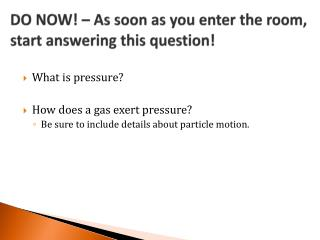DownloadDownload PresentationDO NOW! – As soon as you enter the room, start answering this question!

# DO NOW! – As soon as you enter the room, start answering this question!

Télécharger la présentation## DO NOW! – As soon as you enter the room, start answering this question!

- - - - - - - - - - - - - - - - - - - - - - - - - - - E N D - - - - - - - - - - - - - - - - - - - - - - - - - - -
##### Presentation Transcript

1. DO NOW! – As soon as you enter the room, start answering this question! • What is pressure? • How does a gas exert pressure? • Be sure to include details about particle motion.

2. DO NOW! • Pressure is a force exerted over an area • , a force over an area of a surface • When gas molecules collide with the walls of their container, they exert a force.

3. Units of Pressure • All of these are equal: • 1.00 atm • 101.325 kPa • 760. mmHg • 760. torr You need to memorize these conversions!

4. Units of Pressure • Standard Pressure – the pressure exerted at sea level by dry air • 1.00 atm (atmosphere) • 101.325 kPa (kilopascals) = 1.00 atm • pascal is the SI unit for pressure (1 Pa = 1 ) • 760. mmHg (millimeters of mercury) = 1.00 atm • 760. torr (Torr [named after Evangelista Torricelli, inventor of the barometer]) = 1.00 atm 1.00 atm = 101.325 kPa = 760. mmHg = 760. torr

5. Other units of pressure • 14.7 psi (or lb./in2) (pounds per square inch) • 29.9 inHg (inches of mercury) • 1.01325 bar (1 bar = 100,000 Pa) (Don’t worry about these ones.)

6. Conversions • All of these units measure pressure, so we can convert between all of them: • 2.59 atm  torr • 657 mmHg  kPa

7. Atmospheric Pressure • At sea level, every surface has 1.00 atm (14.7 lb./in2 for reference) of pressure pushing on it at all times. • How does the pressure mat work? • There is no air underneath the mat, so there is no atmospheric pressure underneath it pushing up. • This means there are (theoretically) more than 2,000 lbs. of force pushing down on the mat!

8. Barometer • Measures the pressure of the atmosphere • Atmospheric pressure pushing down on the pool of liquid forces liquid to stay in the tube. • The height of the liquid in the tube measures pressure, regardless of the shape or size of the tube.

9. Manometer • Measures the pressure of a gas. • Compares Pgas to Patm Pgas < Patm Pgas > Patm

10. Partial Pressure • Dalton’s Law of partial pressure • the total pressure of a gas mixture is the sum of the partial pressures of the gases in the mixture. • Total pressure = sum of partial pressures • PT = P1 + P2 + P3 + … + = + =

11. Partial Pressure Problems • A sample of gas contains N2 at 185 torr, Ar at 90. torr, and O2 at 35 torr. What is the total pressure of the gas?

12. Partial Pressure Problems • A sample of gas contains N2 at 185 torr, Ar at 90. torr, and O2 at 35 torr. What percent of the gas is Ar?

13. Partial Pressure Problems • A sample of gas mixture is 35% N2. If the total pressure of the gas mixture is 724 kPa, what is the partial pressure of N2?

14. Collection over Water • Gases are often collected over water • In the test tube where gas is collected, water vapor is also present. • Vapor pressure varies with temperature.

15. Collection over Water • A 30.0 mL sample of gas is collected over water at 20 °C and 101.325 kPa of pressure. What is the pressure of the dry gas alone? Vapor pressure at 20 °C is 2.3 kPa.

16. Exit Slip – You’re not allowed to leave unless you’ve filled this out! • Why was the pressure mat so difficult to lift off the lab bench?